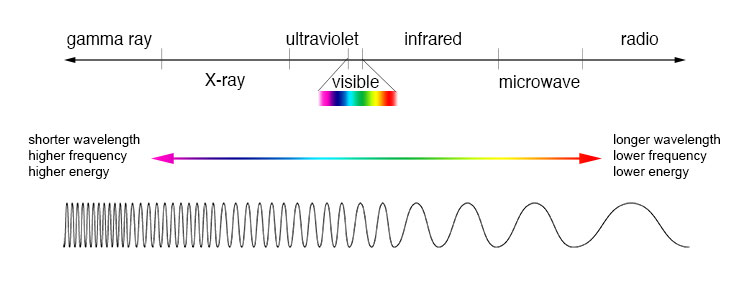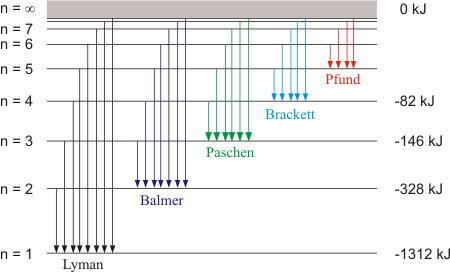## Some Important Characteristics of a WaveA wave is a sort of disturbance which originates from some vibrating source and travels outward as a continuous sequence of alternating crests and troughs. Every wave has five important characteristics, namely, wavelength (λ), frequency (v), velocity (c), wave number(v ¯ )  and amplitude (a).

## What is Electronic Magnetic Radiation?

Ordinary light rays, X–rays,λ–rays, etc. are called electromagnetic radiations because similar waves can be produced by moving a charged body in a magnetic field or a magnet in an electric field. These radiations have wave characteristics and do not require any medium for their propagation.
• Wavelength (λ): The distance between two neighbouring troughs or crests is known as wavelength. It is denoted by l and is expressed in cm, m, nanometers (1 nm =10–9 m) or Angstrom (1 Å=10–10 m).

• Frequency (v): The frequency of a wave is the number of times a wave passes through a given point in a medium in one second. It is denoted by n(nu) and is expressed in cycles per second (cps) or hertz (Hz) 1Hz = 1cps.
The frequency of a wave is inversely proportional to its wave length (λ)
v ∝ 1 / λ  or v = c / λ

• Velocity: The distance travelled by the wave in one second is called its velocity. It is denoted by c and is expressed in cm sec–1.
c = vλ or λ = c / v

• Wave number ( v- ): It is defined as number of wavelengths per cm. It is denoted by v- and is expressed in cm–1.
v= 1 / λ or v- = v / c

• Amplitude: It is the height of the crest or depth of the trough of a wave and is denoted by a. It determines the intensity or brightness of the beam of light.

Wave length (Å)

31014 to 3 ´107

Micro waves

3109 to 3 ´106

Infrared (IR)

6106 to 7600

### Visible

7600 to 3800

Ultra violet (UV)

3800 to 150

X–rays

150 to 0.1

Gamma rays

0.1  to 0.01

Cosmic rays

0.01 to zero

Refer to the following simulation to understand the progression of a wave in proper way

## Electromagnetic SpectrumThe arrangement of the various types of electromagnetic radiation in order of increasing or decreasing wavelengths or frequencies is known as electromagnetic spectrum.## What is Atomic Spectrum?

If the atom gains energy the electron passes from a lower energy level to a higher energy level, energy is absorbed that means a specific wave length is absorbed. Consequently, a dark line will appear in the spectrum. This dark line constitutes the absorption spectrum.

If the atom loses energy, the electron passes from higher to a lower energy level, energy is released and a spectral line of specific wavelength is emitted. This line constitutes the emission spectrum.

Types of Emission Spectra:

• Continuous spectra: When white light from any source such as sun or bulb is analysed by passing through a prism, it splits up into seven different wide bands of colour from violet to red (like rainbow). These colour are so continuous that each of them merges into the next. Hence the spectrum is called as continuous spectrum.

• Line spectra: When an electric discharge is passed through a gas at low pressure light is emitted. If this light is resolved by a spectroscope, It is found that some isolated colored lines are obtained on a photographic plate separated from each other by dark spaces. This spectrum is called line spectrum. Each line in the spectrum corresponds to a particular wavelength. Each element gives its own characteristic spectrum.

• Absorption spectrum: This kind of spectrum has dark band of lines when electromagnetic radiation is passed through a material which absorbs it. The bands corresponding to the energy absorbed by the material appears dark in the spectrum.

• Emission spectrum: This kind of spectrum is observed when the energy absorbed by a material is emitted. When energy is absorbed by a material, its electrons get excited to a higher energy state. When the excited electrons return to their ground state they emit radiation.

## Hydrogen Spectrum

If an electric discharge is passed through hydrogen gas taken in a discharge tube under low pressure, and the emitted radiation is analyzed with the help of spectrograph, it is found to consist of a series of sharp lines in the UV, visible and IR regions. This series of lines is known as line or atomic spectrum of hydrogen. The lines in the visible region can be directly seen on the photographic film.

Each line of the spectrum corresponds to a light of definite wavelength. The entire spectrum consists of six series of lines, each series, known after their discoverer as the Balmer, Paschen, Lyman, Brackett, Pfund and Humphrey series. The wavelength of all these series can be expressed by a single formula.

1/ λ  v- = R  (1/ n12  - 1/n2

Where,  = wave number

λ = wave length

R = Rydberg constant (109678 cm–1)

n1 and n2 have integral values as follows

 Series n1 n2 Main spectral lines Lyman 1 2,3,4, etc Ultra – violet Balmer 2 3,4,5 etc Visible Paschen 3 4,5,6  etc Infra – red Barckett 4 5,6,7 etc Infra – red Pfund 5 6,7,8, etc Infra – redNote: All lines in the visible region are of Balmer series but reverse is not true. i.e., all Balmer lines will not fall in visible region.The pattern of lines in atomic spectrum is characteristic of hydrogen.

## Related Resources

To read more, Buy study materials of Structure of Atom comprising study notes, revision notes, video lectures, previous year solved questions etc. Also browse for more study materials on Chemistry here